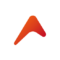# 駭客入侵(修正) – 在 Magento 2 後台 Form 表單取得 categories 功能4 years agoNo Comments```const REPEATER = "__";
const PREFIX_END = '';

\$categories = \$this->getCategoryCollection(true, false, false, false);

private function _toArray()
{
\$categories = \$this->getCategoryCollection(true, false, false, false);

\$catagoryList = array();
foreach (\$categories as \$key => \$category) {
\$prefix = str_repeat(self::REPEATER, (\$category->getLevel()-1) * 1) . self::PREFIX_END;
\$catagoryList[\$category->getEntityId()] = \$prefix. \$category->getName();
}

return \$catagoryList;
}```

Item 1

__Item 2

____Item 3

```private function _toArray()
{
\$categories = \$this->getCategoryCollection(true, false, false, false);
\$catagoryList = array();
\$i = 0;
foreach (\$categories as \$key => \$category) {
if(\$i == 0){
\$rootLevel = \$category->getLevel();
//this is the level of our root category
}
if(\$category->getLevel() == \$rootLevel){
\$i++;
continue;
//we want to skip the root categories like so
}
if(\$category->getLevel()-1 < 0){
\$i++;
continue;
//we want to make sure that we aren't going to break the str_repeat
}
\$z = \$rootLevel + 1;
//we want to do this so we don't have any underscores on our top level - that would be even uglier!!
\$prefix = str_repeat(self::REPEATER, (\$category->getLevel()-\$z) * 1) . self::PREFIX_END;
\$catagoryList[\$category->getEntityId()] = \$prefix. \$category->getName();
\$i++;
}

return \$catagoryList;
}```

```\$("option").each(function() {
var text = \$(this).text();
text = text.replace(/\_/g, "\u2003");
\$(this).text(text);
});```

0### 推薦文章

##### Categories:
Magento2 開源版MagentoMagento2 商業版後端開發網站技術與設計